# NetWeaver: Graphic Presentation of Complex Genomic and Network Data Analysis

## Scope

This guide provides an overview of using the `R` package `NetWeaver` for visualizing complex structural features of network modules. `NetWeaver` is motivated towards developing a simple and flexible pipeline for visualizing the complex features of enrichment and correlation of gene coexpression network modules. While circos style 2D track plot is one natural choice for such practice, existing packages are designed primarily for handling genome structure and intervals. They are either too complicated to use, requiring certain level of knowledge of scripting, or limited in applications to only genomic structure data. To address these issues, particularly extend beyond applications in genomic structure data, `NetWeaver` offers a lightweight implementation of circular track plot, providing simple and flexible R function utilities and pipelines to generate circular images for visualizing different types of structure/relationship data.

## Citing `NetWeaver`

The original version of this package was developed for Figure 7 of Wang et al (2016) Genome Medicine 8:104, which illustrates more than 20 properties for 50 coexpression network modules with a circular track plot. Please try to cite the paper and package when you use results from this software in a publication:

1. Wang M, Roussos P, McKenzie A, Zhou X, Kajiwara Y, Brennand K, DeLuca GC, Crary JF, Casaccia P, Buxbaum J et al. 2016. Integrative Network Analysis of Nineteen Brain Regions Identifies Molecular Signatures and Networks Underlying Selective Regional Vulnerability to Alzheimer`s Disease. Genome Medicine 8: 104.

2. Wang M, Zhang B, 2016. NetWeaver: Graphic Presentation of Complex Genomic and Network Data Analysis [computer software]. doi:10.7303/syn7898789.

## Case study: gene coexpression network modules

We demonstrate the utility of the package through visualizing complex properties of coexpression network modules. Before doing any plotting, let us first define gradient colors which will be used later on:

``````colfuncBlue=colorRampPalette(c("white", "blue"))
colfuncBrown=colorRampPalette(c("white", "brown"))
colfuncHeat=function(n) rev(heat.colors(n))
nCol=50 #number of colors in a gradient``````

``````library("NetWeaver")
data("Modules")``````

The loaded data object is a data.frame called `Modules`. Each row is a module (i.e. a gene cluster), with the module id in the first column. The second column is ranking score computed in regard to disease relevance. The next 4 columns are coefficients of module-trait correlations. The rest columns are P values of enrichment for various gene signatures. To check the structure of the imported object, we can use command:

``str(Modules)``

In this tutorial, we are going to plot the columns of `Modules` into text/barchart/histogram/heat-map using a circos style circular track diagram. Circos plot was originally developed for visualizing genomic strcture data, in which the features are positional variables contained within special sequence structure called chromosomes. Naturally, the basic building blocks of genomic data in a circos plot are chromosomes. With gene coexpression network modules such as in the present sample data `Modules`, the structural containers are modules (i.e. gene clusters) and the features are module properties such as correlation coefficients and enrichment P values in the columns. Though a gene module is different from a chromosome, it makes the circular track plot much easier by treating a module as a hypothetical chromosome.

Using the current package `NetWeaver`, the first step of plotting a circular track diagram is to initialize plotting parameters from chromosome cytoband configurations. The format of chromosome cytoband data is basically a data.frame of chromosomal position ordered cytobands, with columns: Chr, Start, End. A brief explaination of the cytoband data format is given in the help text of function `rc.initialize`:

``?rc.initialize``

With gene coexpression modules, we can create hypothetical cytoband data in a way like:

``cyto=data.frame(Chr=Modules\$id, Start=1, End=100, BandColor="black", stringsAsFactors=FALSE)``

Here we set module id as the chromsome name. Each hypothetical chmosome has a size of 100 with Start position of 1 and End position of 100. Initialize the plot parameters:

``rc.initialize(cyto, num.tracks=36, params=list(chr.padding=0.1))``

through which we specify the canvas to have 36 tracks, which are larger than the number of columns of input data in order to leave sufficient sapce in the middle of the diagram. The plot parameters can be retrieved by

``(params=rc.get.params())``
``````## \$chr.padding
##  0.1
##
## \$default.tracks
##  4
##
## \$default.track.height
##  0.15
##
##  1
##
## \$default.x.length
##  1
##
## \$color.line
##  "black"
##
## \$color.hist
##  "red"
##
##  0.1
##
## \$num.tracks
##  36
##
## \$track.height
##  0.15
##
##  6.28
##
## \$sector.degree
##  6.283185
##
## \$Layout
##  "circular"``````

Please see `?rc.initialize` for an explanation of the parameters. We can change the parameter settings through function `rc.reset.params`. For example, assume we want to use a new color for histogram (default color `red`), the following command will reset the histogram color:

``````params\$color.hist <- "#A349A4"
rc.reset.params(params)``````

It must be noted that change of parameter will only affect the subsequent operations but not what is already done.

Prepare a new canvas (graphics frame):

``rc.plot.area(size=0.9)``

Argument `size` has a value of between 0 to 1, specifying the effective size of the circle plot area in the current canvas. The smaller the value of `size`, the larger the blank area around the circle plot. As canvas is ready, we can plot ideogram of chromosome cytoband in the out-most first two tracks:

``````chrom.alias=1:nrow(cyto)
names(chrom.alias)=cyto\$Chr
rc.plot.ideogram(track.ids=1:2, plot.band=FALSE, plot.chromosome.id=TRUE, cex.text=1.0, chrom.alias=chrom.alias, track.border=NA, polygon.border=NA)``````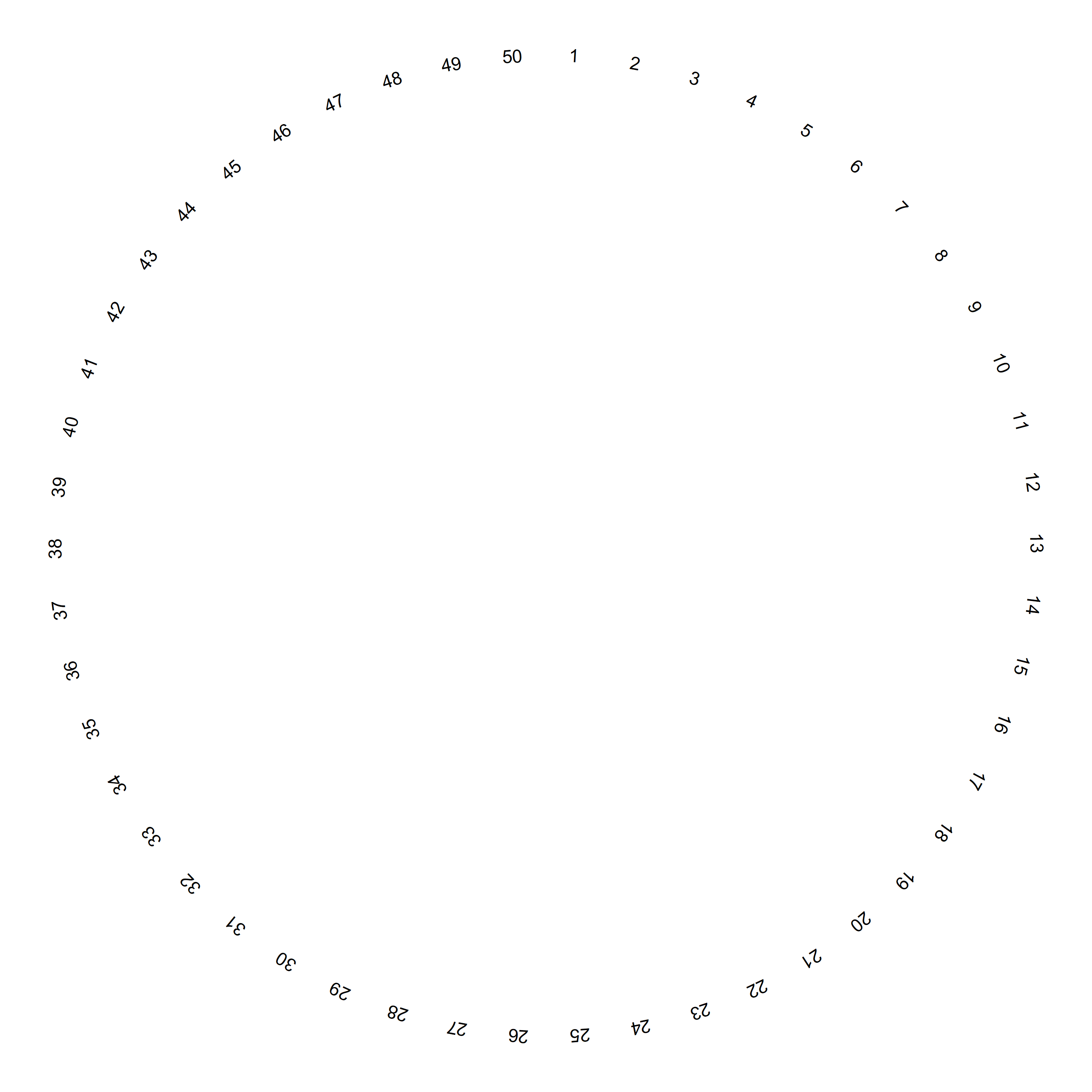Figure 1. Ideogram

`plot.band=FALSE` hence ideogram cytoband in track 2 is not shown. Argument `chrom.alias` is used to display customized container id other than the original chromosome (module) name.

Next to ideogram, let us plot module ranking score using a barchart in tracks 4 to 2:

``````Rank=data.frame(cyto[,c("Chr","Start","End")], Score=Modules\$Score, stringsAsFactors=FALSE)
rc.plot.histogram(Rank, track.id=4, data.col="Score", fixed.height=FALSE, custom.track.height=params\$track.height*3, track.border=NA, polygon.border=NA)``````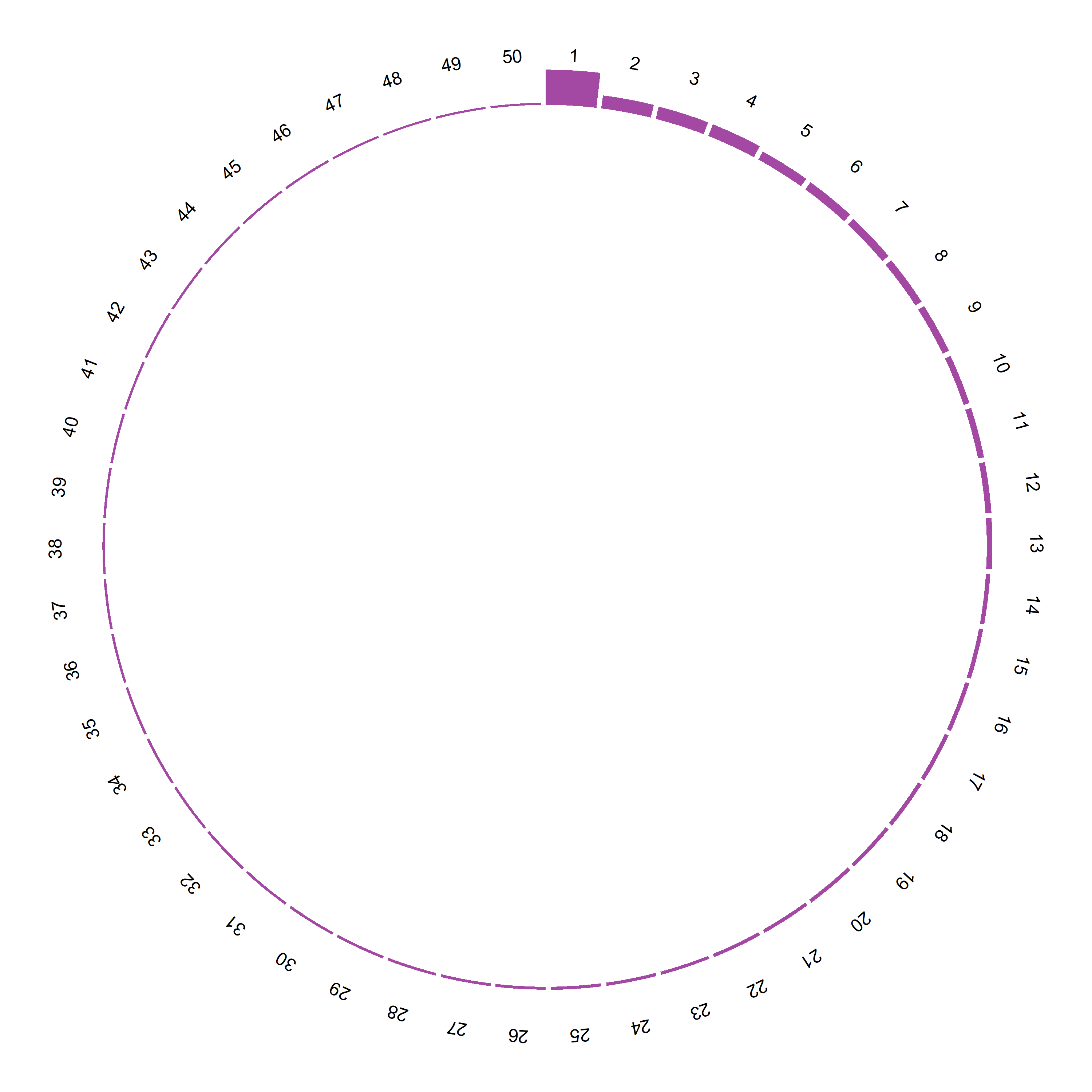Figure 2. Module ranking

Note that we set a customized track height as three times the default track height so that the barchart can make use of space sapnning tacks 2-4 beggining from track 4.

Starting from track 5, we will plot module-trait correlations in multiple tracks:

``````eigenCorCols=colnames(Modules)[grep("Rho",colnames(Modules))] #select columns with pattern Rho
maxCorr=1 #set maximum correlation coefficient
track.border="#999999"
track.color="white"
track.num=4
for(eigenCorCol in eigenCorCols){
track.num <- track.num+1
data.col <- 4
color.col <- 5
HistData=data.frame(Chr=Modules\$id,Start=1,End=100,Data=Modules[,eigenCorCol],
stringsAsFactors=FALSE)
#plot positive correlation
HistData1=HistData[HistData\$Data > 0,]
HistData1\$col=colfuncBrown(nCol)[pmax(1,floor(HistData1[,data.col]*nCol/maxCorr))]
rc.plot.histogram(HistData1, track.num, data.col, color.col=color.col,
fixed.height=TRUE, track.color=track.color, track.border=track.border, polygon.border=NA)
#plot negative correlation
HistData2=HistData[HistData\$Data <= 0,]
HistData2\$Data=abs(HistData2\$Data)
HistData2\$col=colfuncBlue(nCol)[pmax(1,floor(HistData2[,data.col]*nCol/maxCorr))]
rc.plot.histogram(HistData2, track.num, data.col, color.col=color.col, fixed.height=TRUE,
track.border=track.border, polygon.border=NA)
}``````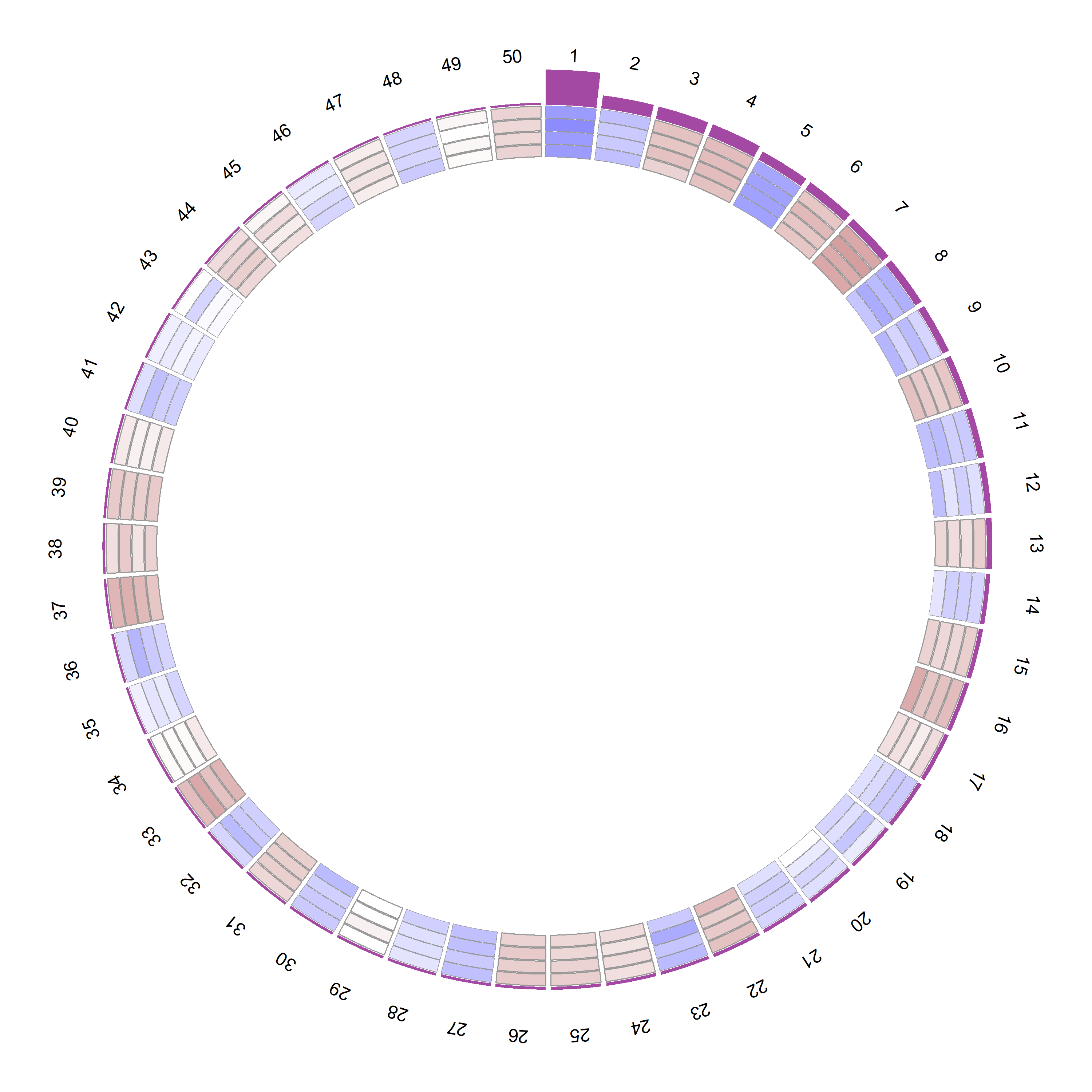Figure 3. Correlation coeffcients

Since we use gradient colors to show the strength of correlations, it is helpful to add a color gradient legend to map colors to correlation coefficient values. For this purpose, I have designed a function `grColLegend` to plot color legend at given x,y-coordinates:

``````y.cor=rc.get.coordinates(1,1,1)\$y-1
bht=0.6
bwt=0.2
rc.plot.grColLegend(x.cor, y.cor, colfuncBrown(nCol), at=c(1,floor(nCol/2),nCol),
legend=signif(c(0,maxCorr/2,maxCorr),2), title=expression(italic(r)),
width=bwt,height=bht, cex.text=0.8)
rc.plot.grColLegend(x.cor, y.cor-bht, rev(colfuncBlue(nCol)), at=c(0,floor(nCol/2)),
legend=signif(c(-maxCorr,-maxCorr/2),2), title="", width=bwt, height=bht, cex.text=0.8)``````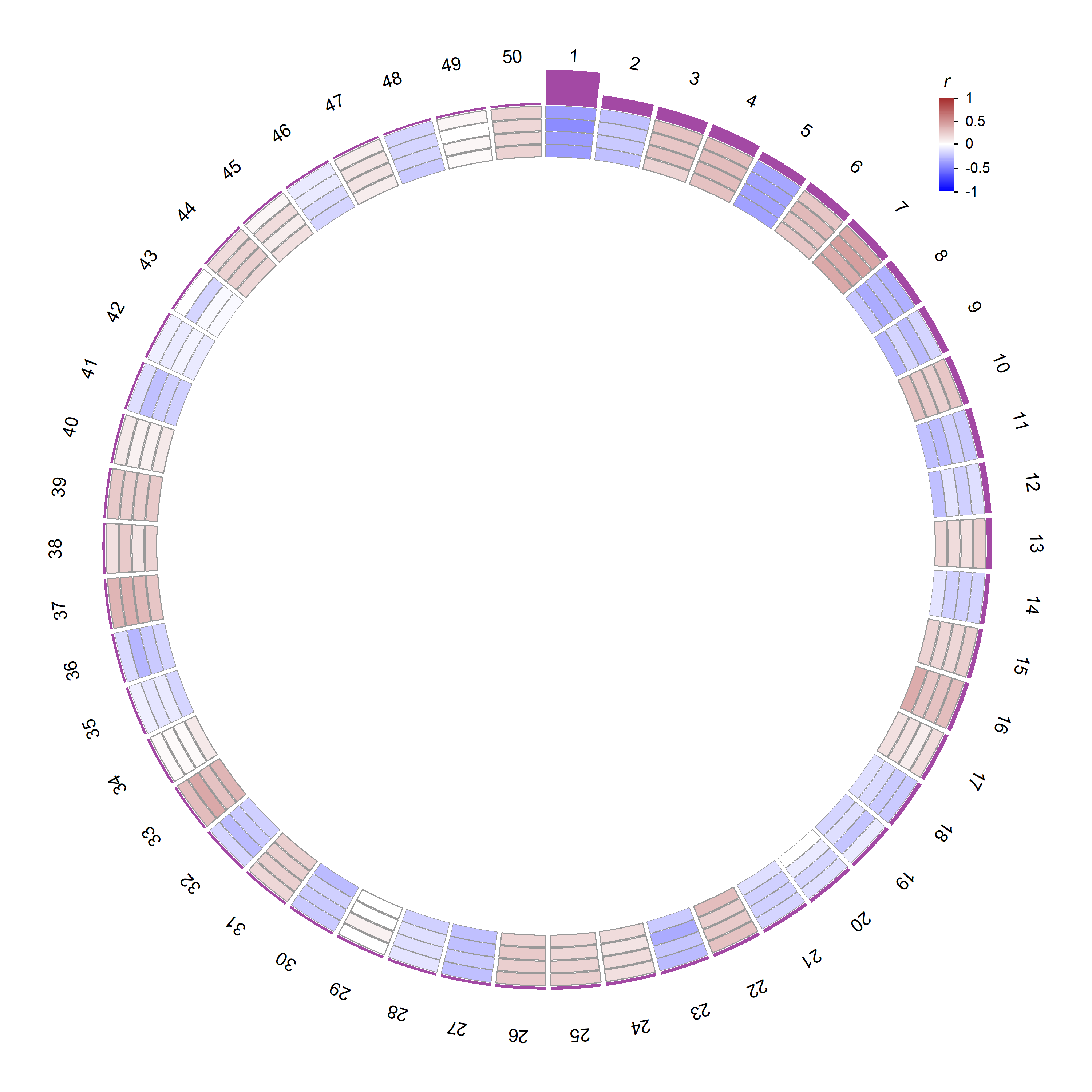Figure 4. Color gradient legend for correlations

Next, we will plot P value significance of enrichment for gene signatures. We can use function `rc.plot.histogram` to plot the data track by track as for plotting correlations. But here we will use the `rc.plot.heatmap` function to plot multiple tracks of heat-map at once:

``````track.num=track.num+1
heatmapData=t(Modules[,grep("Enrichment.*.Pvalue",colnames(Modules))])
colnames(heatmapData)=Modules\$id
#convert P value to -log10 scale
heatmapData[,]=as.integer(-log(heatmapData+1.0e-320,base=10))
#cap the maximum -log10 P value so that there is a richer color pattern
maxLogPval=25
heatmapData[heatmapData>maxLogPval]=maxLogPval
#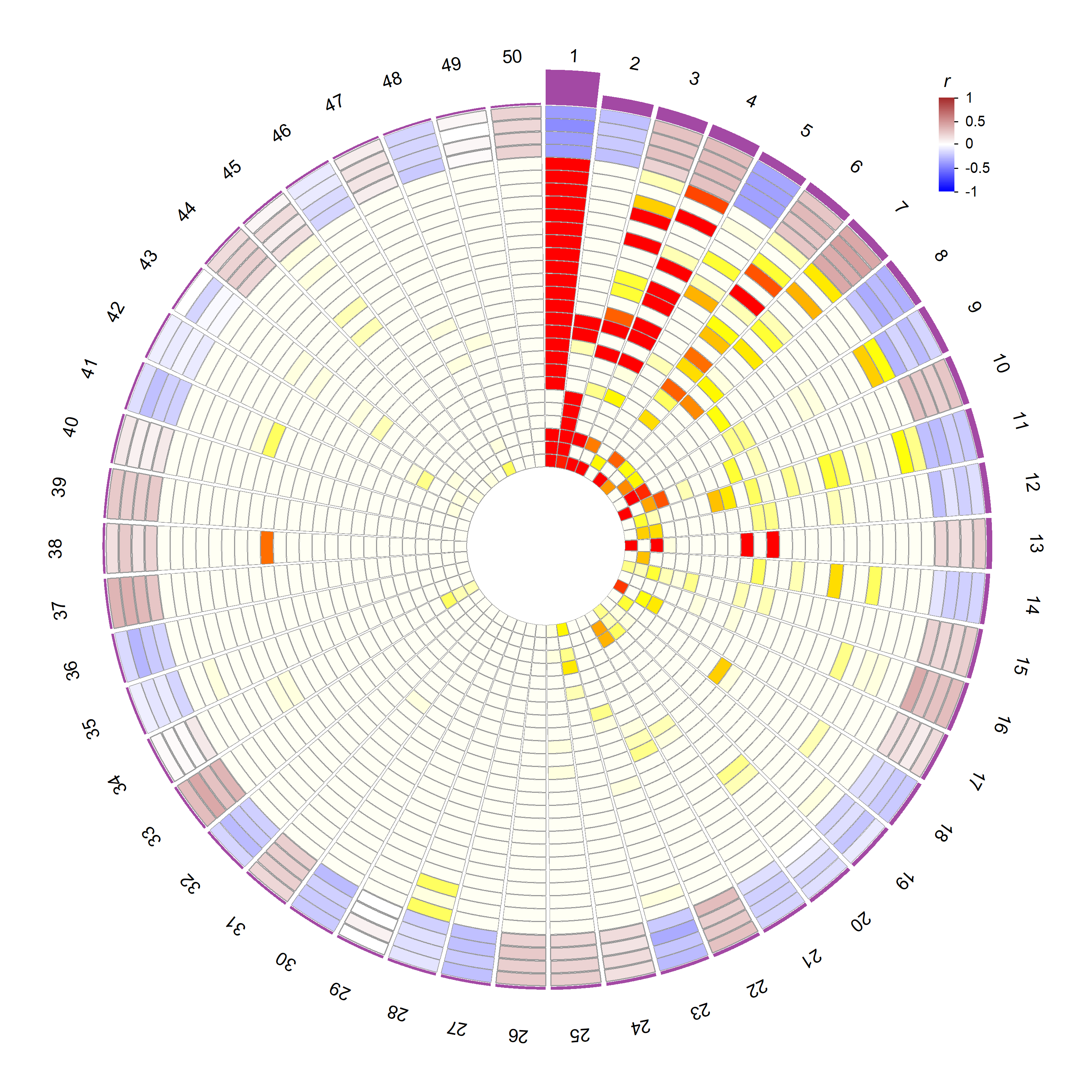``````rc.plot.grColLegend(x.cor+0.8, y.cor-bht, colfuncHeat(nCol), at=c(1,floor(nCol/2),nCol),